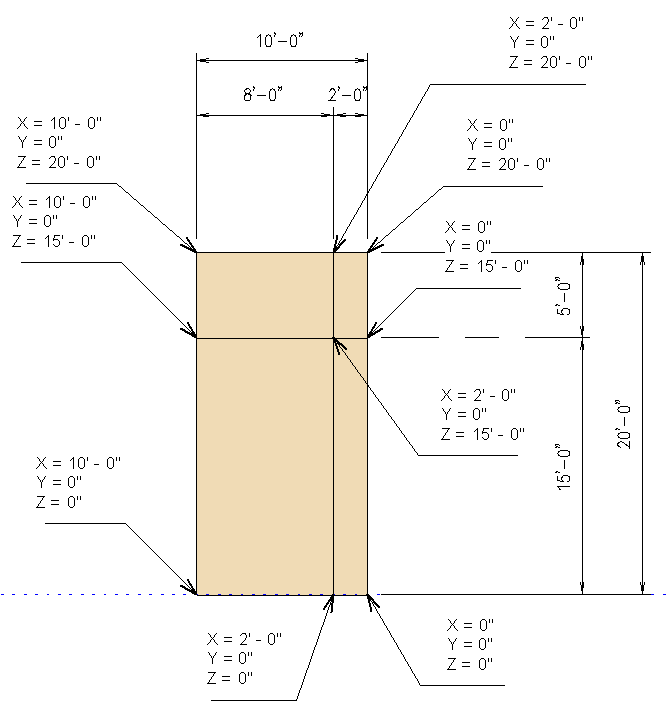### Iterating Over an Unordered Set Property

Here is a simple question that recently came up on iterating over the panel cells in a curtain grid:

Question: I'm noticing some odd behaviour when dealing with CurtainCell.CurveLoops and CurtainCell.PlanarizedCurveLoops taken from CurtainGrid.Cells. It appears that one of the loops is missing and another one of the loops is duplicated.

I am iterating over the cells using the following code and displaying a message listing the loop edge coordinates:

```Dim cell As CurtainCell

For i As Integer = 0 To cg.Cells.Size - 1
cell = cg.Cells(i)

msg += vbCrLf + vbCrLf + "i=" + i.ToString
msg += vbCrLf + "CurveLoops"

Dim iCounter As Integer = 0

For Each cArr As CurveArray In cell.CurveLoops

For Each c As Curve In cArr

iCounter += 1

msg += vbCrLf + iCounter.ToString _
+ ". " + Util.CurveToString(c)

Next

Next

Next

MsgBox(msg)
```

Here are the cells I am examining:The resulting dialogue box displays the cell curve loop coordinates like this:As you can see, one loop is missing and the other is duplicated.

Is this normal behaviour?

Also, I noticed that every time I run the query the order in which they are received changes.

Answer: First, I converted your code to iterate using for each instead:

```Dim cg As CurtainGrid = w.CurtainGrid

Dim msg As String = "# Cells = " _
+ cg.Cells.Size.ToString

Dim cell As CurtainCell
Dim i As Integer = 0

For Each cell In cg.Cells

msg += vbCrLf + vbCrLf + "i=" + i.ToString
msg += vbCrLf + "CurveLoops"

Dim iCounter As Integer = 0

For Each cArr As CurveArray In cell.CurveLoops

For Each c As Curve In cArr

iCounter += 1

msg += vbCrLf + iCounter.ToString _
+ ". " + Util.CurveToString(c)

Next

Next

i += 1

Next

MsgBox(msg)
```

Lo and behold, the problem is resolved, and here are the correct curve loops and their coordinates:The problem has nothing to do with whether you use For and indexing or For Each, though. For Each loops operate in the same manner as a For i = loop. The indexing is provided by extension methods from Linq.

The problem is due to the fact that each call to CurtainGrid.Cells returns a new set.

The set returned is unordered, so there is no guarantee that one set will have its elements in the same order as another.

In your original code, you were calling cg.Cells(i) and thus requesting a new differently ordered set to be returned on each iteration step of the loop.

You can get a reliable indexed access if you obtain and store one single reference to the set once, rather than accessing it in each iteration within the loop:

```Dim cgSet As CurtainCellSet = cg.Cells

For i As Integer = 0 To cgSet.Size - 1
Dim cell As CurtainCell = cgSet(i)

...
```

I have seen several examples in the Revit API where you have to be very aware of the effect of calling a property and storing the result.

In most cases so far, the issue has been calling a property, storing the result in a variable, modifying the value of that variable, and expecting the underlying property to have changed. That is not the case, since the variable just stores a copy of the original value, and the original is not modified by modifying the copy stored in the variable.

In this case, it is the other way round, sort of: each call to the property returns a different result, so we have to store the property value from one single call in a constant variable to ensure that it remains unchanged during the iteration process.